# ECE264: Huffman Coding

The description is mainly taken from Professor Vijay Raghunathan. In this assignment, you will utilize your knowledge about priority queues, stacks, and trees to design a file compression program and file decompression program (similar to zip and unzip). You will base your utilities on the widely used algorithmic technique of Huffman coding, which is used in JPEG compression as well as in MP3 audio compression.

## ASCII coding

Many programming languages use ASCII (which stands for American Standard Code for Information Interchange) coding to represent characters. In ASCII coding, every character is encoded (represented) with the same number of bits (8-bits) per character. Since there are 256 different values that can be represented with 8-bits, there are potentially 256 different characters in the ASCII character set, as shown in the ASCII character table available at http://www.asciitable.com/.

Let us now look at a simple example of ASCII encoding of characters. Using ASCII encoding (8 bits per character) the 13-character string "go go gophers" requires 13 * 8 = 104 bits. The table below shows how the coding works.

 Character ASCII code 8-bit binary value Space 32 00100000 e 101 01100101 g 103 01100111 h 104 01101000 o 111 01101111 p 112 01110000 r 114 01110010 s 115 01110011

The given string would be written as the following stream of bits (the spaces would not be written, just the 0's and 1's)

01100111 01101111 00100000 01100111 01101111 00100000 01100111 01101111 01110000 01101000 01100101 01110010 01110011

Note that we assume that for each byte, we have the most significant bit on the left and the least significant bit on the right for this PE/PA. It does not really matter except that the compression and decompression programs must follow the same bit ordering.

## From ASCII coding towards Huffman coding

Next, let us see how we might use fewer bits using a simpler coding scheme. Since there are only 8 different characters in "go go gophers", it is possible to use only 3-bits to encode the 8 different characters. We might, for example, use the coding shown in the table below (keep in mind that other 3-bit encodings are also possible).

 Character Code Value 3-bit binary value g 0 000 o 1 001 p 2 010 h 3 011 e 4 100 r 5 101 s 6 110 Space 7 111

Now the string "go go gophers" would be encoded as: 000 001 111 000 001 111 000 001 010 011 100 101 110. As you can see, by using three bits per character instead of eight bits per character that ASCII uses, the string "go go gophers" uses a total of 39 bits instead of 104 bits.

However, even in this improved coding scheme, we used the same number of bits to represent each character, irrespective of how often the character appears in our string. Even more bits can be saved if we use fewer than three bits to encode characters like g, o, and space that occur frequently and more than three bits to encode characters like e, h, p, r, and s that occur less frequently in "go go gophers". This is the basic idea behind Huffman coding: to use fewer bits for characters that occur more frequently. We'll see how this is done using a tree data structure that stores the characters as its leaf nodes, and whose root-to-leaf paths provide the bit sequence used to encode the characters.

### Towards a Coding Tree

Using a binary tree for coding, all characters are stored at the leaves of a tree. A left-edge is numbered 0 and a right-edge is numbered 1. The code for any character/leaf node is obtained by following the root-to-leaf path and concatenating the 0's and 1's. The specific structure of the tree determines the coding of any leaf node using the 0/1 edge convention described. As an example, the tree below on the right yields the coding shown on the left.

 Character Binary code '  ' 101 'e' 1100 'g' 00 'h' 1101 'o' 01 'p' 1110 'r' 1111 's' 100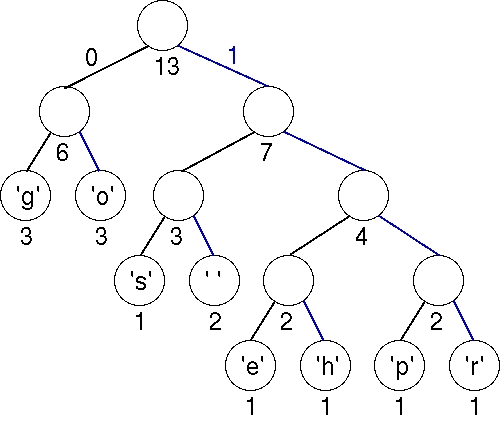Using this coding, "go go gophers" is encoded (again, spaces would not appear in the bit-stream) as: 00 01 101 00 01 101 00 01 1110 1101 1100 1111 100. This is a total of 37 bits, two bits fewer than the improved encoding in which each of the 8 characters has a 3-bit encoding! The bits are saved by coding frequently occurring characters like 'g' and 'o' with fewer bits (here two bits) than characters that occur less frequently like 'p', 'h', 'e', and 'r'.

To decode a given stream that has been coded by the given tree, start at the root of the tree, and follow a left-branch if the next bit in the stream is a 0, and a right branch if the next bit in the stream is a 1. When you reach a leaf, write the character stored at the leaf, and start again at the top of the tree. The bit stream 10011101101110011111100 yields right-left-left to the letter 's', followed (starting again at the root) with right-right-right-left to the letter 'p', followed by right-right-left-right to the letter 'h'. Continuing thus yields a decoded string “sphere”.

### Prefix codes

When all characters are stored in leaves, and every interior (non-leaf) node has two children, the coding induced by the 0/1 convention outlined above satisfies a very important property called the prefix property which states that no bit-sequence encoding of a character is the prefix of the bit-sequence encoding of any other character. This makes it possible to decode a bitstream using the coding tree by following root-to-leaf paths. The tree shown above for "go go gophers" satisfies this prefix property and is an optimal tree. There are other trees that use 37 bits; for example you can simply swap any sibling nodes and get a different encoding that uses the same number of bits. Next, we look at an algorithm for constructing such an optimal tree. This algorithm is called Huffman coding, and was invented by David A. Huffman in 1952 when he was a Ph.D. student at MIT.

## Huffman Coding

In the previous section we saw examples of how a stream of bits can be generated from an encoding. We also saw how the tree can be used to decode a stream of bits. We'll discuss how to construct the tree here using Huffman's algorithm.

We'll assume that associated with each character is a weight that is equal to the number of times the character occurs in a file. For example, in the string "go go gophers", the characters 'g' and 'o' have weight 3, the space has weight 2, and the other characters have weight 1. When compressing a file we'll need to first read the file and calculate these weights. Assume that all the character weights have been calculated. Huffman's algorithm assumes that we're building a single tree from a group (or forest) of trees. Initially, all the trees have a single node containing a character and the character's weight. Iteratively, a new tree is formed by picking two trees and making a new tree whose child nodes are the roots of the two trees. The weight of the new tree is the sum of the weights of the two sub-trees. This decreases the number of trees by one in each iteration. The process iterates until there is only one tree left. The algorithm is as follows:

1. Begin with a forest of trees. All trees have just one node, with the weight of the tree equal to the weight of the character in the node. Characters that occur most frequently have the highest weights. Characters that occur least frequently have the smallest weights.
1. Repeat this step until there is only one tree:

Choose two trees with the smallest weights; call these trees T1 and T2. Create a new tree whose root has a weight equal to the sum of the weights T1 + T2 and whose left sub-tree is T1 and whose right sub-tree is T2.

1. The single tree left after the previous step is an optimal encoding tree.

We shall use the string "go go gophers" as an example. Initially we have the forest shown below. The nodes are shown with a weight that represents the number of times the node's character occurs in the given input string/file.We pick two minimal nodes. There are five nodes with the minimal weight of 1. In this implementation, we maintain a priority queue with items arranged according to their weights. When two items have the same weight, a leaf node (i.e., a node associated with an ASCII character) is always ordered first. If both nodes are leaf nodes, they are ordered according to their ASCII coding. If both nodes are non-leaf nodes, they are ordered according to the creation times of the nodes. We always pick the first two items in the priority queue, namely, nodes for characters 'e' and 'h'. We create a new tree whose root is weighted by the sum of the weights chosen. The order of the nodes in the priority queue also determines the left and right child nodes of the new root. We now have a forest of seven trees as shown here. Although the newly created node has the same weight as Space, it is ordered after Space in the priority queue because Space is an ASCII character.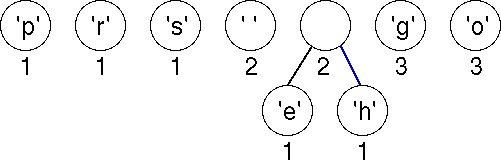Choosing the first two (minimal) nodes in the priority queue yields another tree with weight 2 as shown below. There are now six trees in the forest of trees that will eventually build an encoding tree.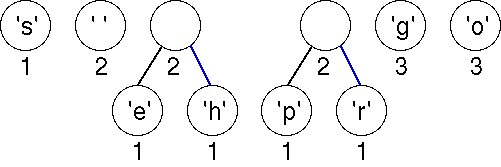Again we must choose the first two (minimal) nodes in the priority queue. The lowest weight is the 'e'-node/tree with weight equal to 1. There are three trees with weight 2; the one chosen corresponds to an ASCII character because of the way we order the nodes in the priority queue. The new tree has a weight of 3, which will be placed last in the priority queue according to our ordering strategy.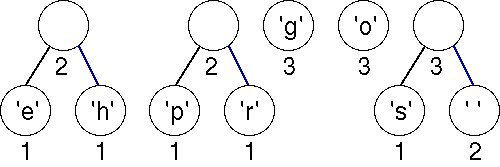Now there are two trees with weight equal to 2. These are joined into a new tree whose weight is 4. There are four trees left, one whose weight is 4 and three with a weight of 3.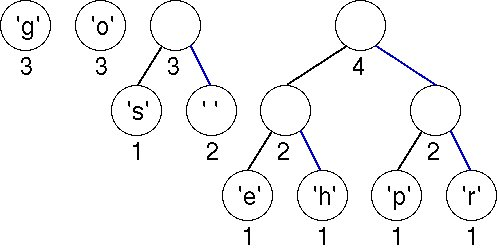The first two minimal (weight 3) trees in the priority queue are joined into a tree whose weight is 6. There are three trees left.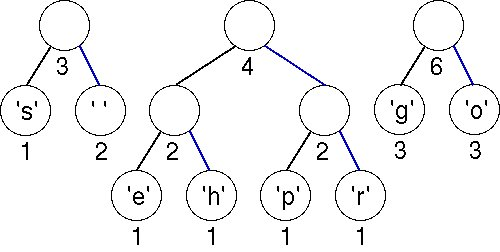The minimal trees have weights of 3 and 4; these are joined into a tree with weight 7.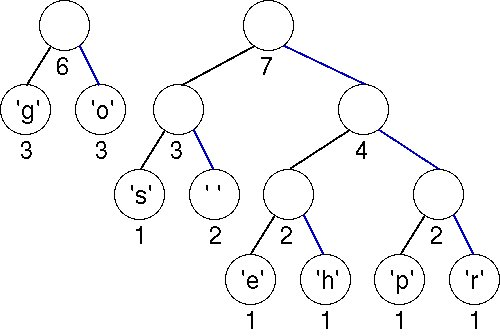Finally, the last two trees are joined into a final tree whose weight is 13, the sum of the two weights 6 and 7. This tree is the tree we used to illustrate Huffman coding above. Note that you can easily come up with an alternative optimal tree by using a different ordering strategy to order trees of the same weights. In that case, the bit patterns for each character are different, but the total number of bits used to encode "go go gophers" is the same.We now show another tree to compress the string "streets are stone stars are not" optimally. To encode "streets are" we would have the following bits: 1110001111011000111101010011110.

Another Huffman Tree/Table Example

 Character Binary value '  ' 101 'a' 010 'e' 110 'n' 1000 'o' 1001 'r' 011 's' 111 't' 00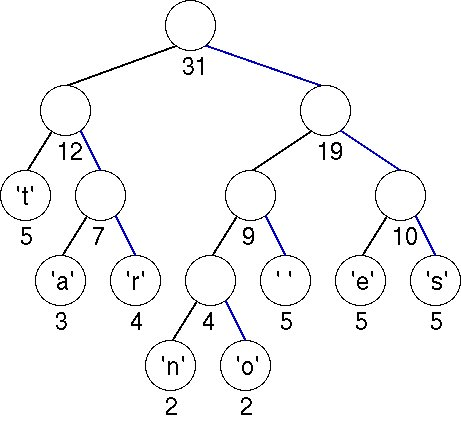It is important to note that you cannot use the tree built for the string "go go gophers" to decode the bitstreams obtained from the encoding of "streets are stone stars are not" as the encoding is performed using a different tree.

## Implementing/Programming Huffman Coding

In this section we'll see the basic programming steps in implementing Huffman coding. There are two parts to an implementation: a compression program and a decompression program. We'll assume these are separate programs, but they actually have many common functions. For this set of programming exercises/assignment, you will deal mainly with decompression. However, to do decompression, we have to understand compression.

### The Compression Program

To compress a file (sequence of characters) you need a table of bit encodings, i.e., a table giving a sequence of bits used to encode each character. This table is constructed from a coding tree using root-to-leaf paths to generate the bit sequence that encodes each character.

A compressed file is obtained using the following top-level steps. These steps will be developed further into sub-steps, and you'll eventually implement a program based on these ideas and sub-steps.

1.     Build a Huffman coding tree based on the number of occurrences of each ASCII character in the file. Build a table of Huffman codes for all ASCII characters that appear in the file.

2.     Read the file to be compressed (the plain file) and process one character at a time. To process each character find the bit sequence that encodes the character using the table built in the previous step and write this bit sequence to the compressed file.

The main challenge here is that when you encode an ASCII character read from the file, the code is typically shorter than 8 bits. However, most systems allow you to write to a file a byte or 8 bits at a time. It becomes necessary for you to accumulate the codes for a few ASCII characters before you write an 8-bit character to the output file.

To compress the string "streets are stone stars are not" for example, we read from the string one character at a time. The code for 's' is 111, we cannot write to the file yet. We read the next character 't', whose code is 00. Again, we cannot write to the output file because the total number of bits is only 5. Now, we read the next character 'r', whose code is 011. The compression program can now print a character of bit pattern 11100011 to the output file.

Again, a reminder that we use the bit ordering in a byte to be one where the most significant bit is on the left and the least significant bit is on the right.

Continuing with the process, the program would have to read 'eet', whose combined code is '11011000', before printing the 8-bit combined code to the output file. When the program reads the next three characters 's a', the combined code is '111101010'. As the combined code has one more than 8 bits, the program should print the first 8 bits to the output file, and combine the remaining bit with the next characters. You may also encounter codes that are longer than 8 bits. You would have to split such codes into 8-bit bytes.

What happens when you reach the end of the file, and you have not accummulated 8 bits for printing. All you have to do is pad the accummulated bits with enough 0's and print it to the output file.

We will show you the header information that a compression program that I have written provides at the beginning of the compressed file so that the decompression program can correctly decode the compressed file.

You must store some initial information in the compressed file that will be used by the decompression/unhuffing program. Essentially, you must store the tree that is used to compress the original file. This is because the decompression program needs this exact same tree in order to decode the data. We will refer to the topology as the header information. At the beginning of the compressed, there are three unsigned integers:

·       The total number of characters in the compressed file, as a 4-byte unsigned integer.

·       The total number of characters storing the header information, i.e., the topology of the Huffman coding tree, as a 4-byte unsigned integer.

·       The total number of characters in the original uncompressed file, as a 4-byte unsigned integer.

Following the three unsigned integers, we store the header information or the topology of the Huffman coding tree, and then followed by the encoding of the original text using Huffman codes. We have described the encoding of the original text in the earlier sections. Now, we focus on how the topology of the Huffman coding tree is stored.

To store the tree at the beginning of the file, we use a post-order traversal, writing each node visited. When you encounter a leaf node, you write a 1 followed by the ASCII character of the leaf node. When you encounter a non-leaf node, you write a 0. To indicate the end of the Huffman coding tree, we write another 0.

Consider the string "go go gophers", the header information is "1g1o01s1 01e1h01p1r00000", immediately followed by encoded text. The post-order traversal of the Huffman coding tree gives us "1g1o01s1 01e1h01p1r0000". Another "0" separates the topology from the encoded text.

For the string "streets are stone stars are not", the header information is "1t1a1r001n1o01 01e1s0000", followed by the encoded text.

In these two examples, we use characters 0 and 1 to distinguish between non-leaf and leaf nodes (and 0 to indicate the end of a topology). As there are eight leaf nodes in each of the two examples, there are eight 1's, seven 0's for non-leaf nodes, and another one 0 to indicate that we have reached the end of a topology. This approached used a total of 24 bytes.

The header information can be made more economical if we use bits 0 and 1 to distinguish between non-leaf and leaf nodes, and also to indicate the end of a topology. In these two examples, there will be a total of 10 bytes (8 bytes for the leaf nodes and 2 bytes for all the 0's and 1's). The challenge here is that both the compression and decompression programs would have to handle bits instead of characters. For example, in the bit-based approach, the first 16 bits (or the first 2 bytes) of the header information for encoding the string "streets are stone stars are not" are 10111010 01011000 (note that the space in the bit stream is introduced for better clarity). The ASCII coding for 't' straddles the two bytes, 7 bits in the first byte and 1 bit in the second byte. The second most significant bit in the second byte is a 1, indicating that the next 8 bits is an ASCII character, of which the the most significant 6 bits of the character 'a' is contained in the least significant 6 bits of the second byte.

In the bit-based representation of the Huffman coding tree, the last byte may not contain 8 bits. In this case, we again pad it with 0 bits. Consider the case where the input file uses nine distinct ASCII characters. The number of bits required to represent the Huffman coding tree is 9×8 + 9×2 = 90 bits, which can represented by 12 bytes. In other words, the last byte should contain only two useful bits. The 12 bytes are followed by the encoded text.

### The Decompression or Dehuffing Program

Given a compressed file, which contains the header information, followed by a bit stream corresponding to the encoding of the original file, the decompression program should perform the following tasks:

1.     Build a Huffman coding tree based on the header information. You would probably need the second unsigned integer stored at the beginning of the compressed file to help with the construction.

2.     Read bit-by-bit from the compressed file (starting at the location after the header information). Use the read bit to traverse the Huffman coding tree (0 for left and 1 for right), starting from the root node. When the program reaches a leaf node, print the corresponding ASCII character to the output file. Starts from the root node of the Huffman coding tree when the next bit is read. The program terminates when the number of characters decoded matches the number of characters stored as the third unsigned integer at the beginning of the compressed file.

To construct a Huffman coding tree from the header information, we make use of a stack. When a 1 (bit or character depending on whether we are dealing with a bit-based or character-based representation) is read, we read the next byte and push the corresponding ASCII character onto the stack. When a 0 (bit or character) is read, if the stack contains only one element, we have constructed the entire Huffman coding tree. Otherwise, there must be more than one element in the stack. We create a new node, and pop the top two elements off the stack. We make the first element off the stack the right child of the new node, and the second element off the stack the left child of the new node. After that, we push the newly created node onto the stack.

A challenge is in the reading of a bit from the compressed file. Again, reading from a file occurs at the byte-level. In the decompression program, you have to read an 8-bit ASCII character that straddle two bytes in the compressed file when you want to construct a Huffman coding tree. You also have to read one bit at a time to determine whether you are dealing with a leaf node or a non-leaf node. Of course, you also have to read one bit at a time when you want to decode the compressed file. A strategy is to always read a byte from the compressed file. You also maintain an index to indicate where you are in that byte. For example, when you read in a character, the index should be pointing to the most significant bit (i.e., the 7th bit, assuming that the least significant bit is the 0th bit). After you have processed one bit, the index should be decremented by 1.